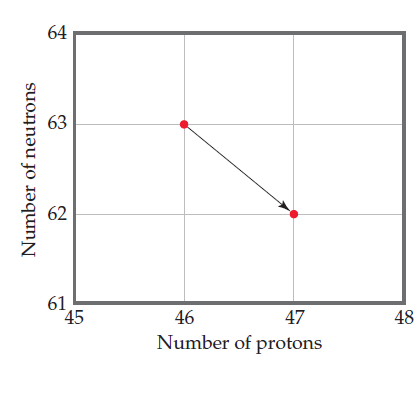×
Get Full Access to Chemistry: The Central Science - 12 Edition - Chapter 21 - Problem 2e
Get Full Access to Chemistry: The Central Science - 12 Edition - Chapter 21 - Problem 2e

×

# Visualizing ConceptsWrite the balanced nuclear equationISBN: 9780321696724 27

## Solution for problem 2E Chapter 21

Chemistry: The Central Science | 12th Edition

• Textbook Solutions
• 2901 Step-by-step solutions solved by professors and subject experts
• Get 24/7 help from StudySoup virtual teaching assistantsChemistry: The Central Science | 12th Edition

4 5 1 288 Reviews
14
0
Problem 2E

VISUALIZING CONCEPTS

Write the balanced nuclear equation for the reaction represented by the diagram shown here. [Section 21.2]Step-by-Step Solution:
Step 1 of 3

Three center two electron bonds The orbitals in H are delocalized over the entire molecule 3 In H + 2 electrons hold the molecule together 3 – this is an example of a three center two electron bond Diborane and electron deficient compounds B H is a compound that you can not draw a reasonable Lewis structure for ! 2 6 XeF and electron excess compounds 2 The electronic structure of XeF and it’s stability can be rationalized by invoking a 3 2 center 4 electron bond

Step 2 of 3

Step 3 of 3

##### ISBN: 9780321696724

This textbook survival guide was created for the textbook: Chemistry: The Central Science, edition: 12. Since the solution to 2E from 21 chapter was answered, more than 464 students have viewed the full step-by-step answer. This full solution covers the following key subjects: balanced, concepts, diagram, equation, here. This expansive textbook survival guide covers 49 chapters, and 5471 solutions. The full step-by-step solution to problem: 2E from chapter: 21 was answered by , our top Chemistry solution expert on 04/03/17, 07:58AM. The answer to “?VISUALIZING CONCEPTSWrite the balanced nuclear equation for the reaction represented by the diagram shown here. [Section 21.2]” is broken down into a number of easy to follow steps, and 17 words. Chemistry: The Central Science was written by and is associated to the ISBN: 9780321696724.

## Discover and learn what students are asking

Unlock Textbook Solution